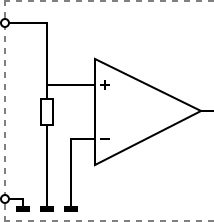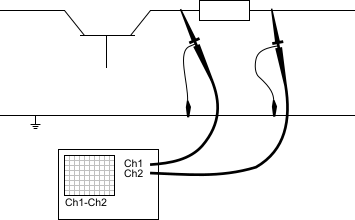# Single ended oscilloscope inputs

Most oscilloscopes are equipped with standard, single ended inputs, which are referenced to ground. This means that one side of the input is always connected to ground and the other side to the point of interest in the circuit under test.Therefore the voltage that is measured with an oscilloscope with standard, single ended inputs is always measured between that specific point and ground.

What if the voltage you're interested in is not referenced to ground? Connecting a standard single ended oscilloscope input to the two points would create a short circuit between one of the points and ground, possibly damaging the circuit and the oscilloscope.

A safe way would be to measure the voltage at one of the two points, in reference to ground and at the other point, in reference to ground and then calculate the voltage difference between the two points. On most oscilloscopes this can be done by connecting one of the channels to one point and another channel to the other point and then use the math function Ch1 - Ch2 in the oscilloscope to display the actual voltage difference.There are some disadvantages to this method:

• to measure one signal, two channels are occupied.
• by using two channels, the measurement error is increased, the errors made on each channel will be combined, resulting in a larger total measurement error.
• The Common Mode Rejection Ratio of this method is relatively low. If both points have a relative high voltage, but the voltage difference between the two points is small, the voltage difference can only be measured in a high input range, resulting in a low resolution.